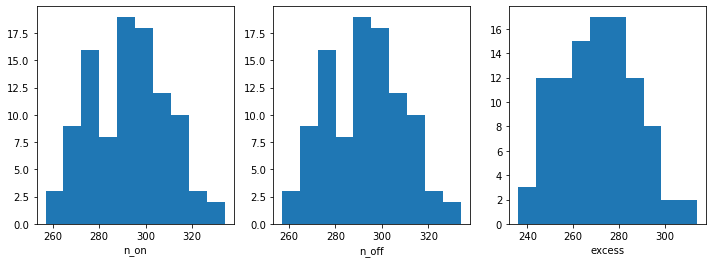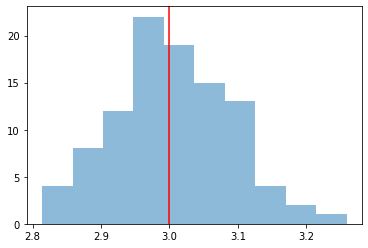This is a fixed-text formatted version of a Jupyter notebook

# Spectrum simulation¶

## Context¶

To simulate a specific observation, it is not always necessary to simulate the full photon list. For many uses cases, simulating directly a reduced binned dataset is enough: the IRFs reduced in the correct geometry are combined with a source model to predict an actual number of counts per bin. The latter is then used to simulate a reduced dataset using Poisson probability distribution.

This can be done to check the feasibility of a measurement, to test whether fitted parameters really provide a good fit to the data etc.

Here we will see how to perform a 1D spectral simulation of a CTA observation, in particular, we will generate OFF observations following the template background stored in the CTA IRFs.

Objective: simulate a number of spectral ON-OFF observations of a source with a power-law spectral model with CTA using the CTA 1DC response, fit them with the assumed spectral model and check that the distribution of fitted parameters is consistent with the input values.

## Proposed approach:¶

We will use the following classes:

• gammapy.datasets.SpectrumDatasetOnOff

• gammapy.datasets.SpectrumDataset

• gammapy.irf.load_cta_irfs

• gammapy.modeling.models.PowerLawSpectralModel

## Setup¶

:

%matplotlib inline
import matplotlib.pyplot as plt

:

import numpy as np
import astropy.units as u
from astropy.coordinates import SkyCoord, Angle
from regions import CircleSkyRegion
from gammapy.datasets import SpectrumDatasetOnOff, SpectrumDataset, Datasets
from gammapy.makers import SpectrumDatasetMaker
from gammapy.modeling import Fit
from gammapy.modeling.models import (
PowerLawSpectralModel,
SpectralModel,
SkyModel,
)
from gammapy.data import Observation
from gammapy.maps import MapAxis


## Simulation of a single spectrum¶

To do a simulation, we need to define the observational parameters like the livetime, the offset, the assumed integration radius, the energy range to perform the simulation for and the choice of spectral model. We then use an in-memory observation which is convolved with the IRFs to get the predicted number of counts. This is Poission fluctuated using the fake() to get the simulated counts for each observation.

:

# Define simulation parameters parameters
livetime = 1 * u.h
pointing = SkyCoord(0, 0, unit="deg", frame="galactic")
offset = 0.5 * u.deg
# Reconstructed and true energy axis
energy_axis = MapAxis.from_edges(
np.logspace(-0.5, 1.0, 10), unit="TeV", name="energy", interp="log"
)
energy_axis_true = MapAxis.from_edges(
np.logspace(-1.2, 2.0, 31), unit="TeV", name="energy", interp="log"
)


:

# Define spectral model - a simple Power Law in this case
model_simu = PowerLawSpectralModel(
index=3.0,
amplitude=2.5e-12 * u.Unit("cm-2 s-1 TeV-1"),
reference=1 * u.TeV,
)
print(model_simu)
# we set the sky model used in the dataset
model = SkyModel(spectral_model=model_simu, name="source")

PowerLawSpectralModel

name     value        unit      min max frozen   error
--------- --------- -------------- --- --- ------ ---------
index 3.000e+00                nan nan  False 0.000e+00
amplitude 2.500e-12 cm-2 s-1 TeV-1 nan nan  False 0.000e+00
reference 1.000e+00            TeV nan nan   True 0.000e+00

:

# Load the IRFs
# In this simulation, we use the CTA-1DC irfs shipped with gammapy.
"\$GAMMAPY_DATA/cta-1dc/caldb/data/cta/1dc/bcf/South_z20_50h/irf_file.fits"
)

:

obs = Observation.create(pointing=pointing, livetime=livetime, irfs=irfs)
print(obs)

Observation

obs id            : 0
tstart            : 51544.00
tstop             : 51544.04
duration          : 3600.00 s
pointing (icrs)   : 266.4 deg, -28.9 deg


:

# Make the SpectrumDataset
dataset_empty = SpectrumDataset.create(
e_reco=energy_axis.edges,
e_true=energy_axis_true.edges,
region=on_region,
name="obs-0",
)
maker = SpectrumDatasetMaker(selection=["aeff", "edisp", "background"])
dataset = maker.run(dataset_empty, obs)

:

# Set the model on the dataset, and fake
dataset.models = model
dataset.fake(random_state=42)
print(dataset)

SpectrumDataset
---------------

Name                            : obs-0

Total counts                    : 290
Total predicted counts          : 294.61
Total background counts         : 22.35

Effective area min              : 8.16e+04 m2
Effective area max              : 5.08e+06 m2

Livetime                        : 3.60e+03 s

Number of total bins            : 9
Number of fit bins              : 9

Fit statistic type              : cash
Fit statistic value (-2 log(L)) : -1742.28

Number of parameters            : 3
Number of free parameters       : 2

Component 0: SkyModel

Name                      : source
Datasets names            : None
Spectral model type       : PowerLawSpectralModel
Spatial  model type       : None
Temporal model type       : None
Parameters:
index                   :   3.000
amplitude               :   2.50e-12  1 / (cm2 s TeV)
reference    (frozen)   :   1.000  TeV



You can see that backgound counts are now simulated

### OnOff analysis¶

To do OnOff spectral analysis, which is the usual science case, the standard would be to use SpectrumDatasetOnOff, which uses the acceptance to fake off-counts

:

dataset_onoff = SpectrumDatasetOnOff.from_spectrum_dataset(
dataset=dataset, acceptance=1, acceptance_off=5
)
dataset_onoff.fake(background_model=dataset.background)
print(dataset_onoff)

SpectrumDatasetOnOff
--------------------

Name                            : obs-0

Total counts                    : 294
Total predicted counts          : 293.46
Total off counts                : 106.00

Total background counts         : 21.20

Effective area min              : 8.16e+04 m2
Effective area max              : 5.08e+06 m2

Livetime                        : 3.60e+03 s

Acceptance mean:                : 1.0

Number of total bins            : 9
Number of fit bins              : 9

Fit statistic type              : wstat
Fit statistic value (-2 log(L)) : 9.46

Number of parameters            : 3
Number of free parameters       : 2

Component 0: SkyModel

Name                      : source
Datasets names            : None
Spectral model type       : PowerLawSpectralModel
Spatial  model type       : None
Temporal model type       : None
Parameters:
index                   :   3.000
amplitude               :   2.50e-12  1 / (cm2 s TeV)
reference    (frozen)   :   1.000  TeV



You can see that off counts are now simulated as well. We now simulate several spectra using the same set of observation conditions.

:

%%time

n_obs = 100
datasets = Datasets()

for idx in range(n_obs):
dataset_onoff.fake(
random_state=idx, background_model=dataset.background,
)
datasets.append(dataset_onoff.copy(name=f"obs-{idx}"))

CPU times: user 752 ms, sys: 20 ms, total: 772 ms
Wall time: 789 ms

:

print(datasets.names)

['obs-0', 'obs-1', 'obs-2', 'obs-3', 'obs-4', 'obs-5', 'obs-6', 'obs-7', 'obs-8', 'obs-9', 'obs-10', 'obs-11', 'obs-12', 'obs-13', 'obs-14', 'obs-15', 'obs-16', 'obs-17', 'obs-18', 'obs-19', 'obs-20', 'obs-21', 'obs-22', 'obs-23', 'obs-24', 'obs-25', 'obs-26', 'obs-27', 'obs-28', 'obs-29', 'obs-30', 'obs-31', 'obs-32', 'obs-33', 'obs-34', 'obs-35', 'obs-36', 'obs-37', 'obs-38', 'obs-39', 'obs-40', 'obs-41', 'obs-42', 'obs-43', 'obs-44', 'obs-45', 'obs-46', 'obs-47', 'obs-48', 'obs-49', 'obs-50', 'obs-51', 'obs-52', 'obs-53', 'obs-54', 'obs-55', 'obs-56', 'obs-57', 'obs-58', 'obs-59', 'obs-60', 'obs-61', 'obs-62', 'obs-63', 'obs-64', 'obs-65', 'obs-66', 'obs-67', 'obs-68', 'obs-69', 'obs-70', 'obs-71', 'obs-72', 'obs-73', 'obs-74', 'obs-75', 'obs-76', 'obs-77', 'obs-78', 'obs-79', 'obs-80', 'obs-81', 'obs-82', 'obs-83', 'obs-84', 'obs-85', 'obs-86', 'obs-87', 'obs-88', 'obs-89', 'obs-90', 'obs-91', 'obs-92', 'obs-93', 'obs-94', 'obs-95', 'obs-96', 'obs-97', 'obs-98', 'obs-99']


Before moving on to the fit let’s have a look at the simulated observations.

:

table = datasets.info_table()

fix, axes = plt.subplots(1, 3, figsize=(12, 4))
axes.hist(table["n_on"])
axes.set_xlabel("n_on")
axes.hist(table["n_on"])
axes.set_xlabel("n_off")
axes.hist(table["excess"])
axes.set_xlabel("excess");Now, we fit each simulated spectrum individually

:

%%time
results = []
for dataset in datasets:
dataset.models = model.copy()
fit = Fit([dataset])
result = fit.optimize()
results.append(
{
"index": result.parameters["index"].value,
"amplitude": result.parameters["amplitude"].value,
}
)

CPU times: user 11.5 s, sys: 158 ms, total: 11.7 s
Wall time: 12.2 s


We take a look at the distribution of the fitted indices. This matches very well with the spectrum that we initially injected, index=2.1

:

index = np.array([_["index"] for _ in results])
plt.hist(index, bins=10, alpha=0.5)
plt.axvline(x=model_simu.parameters["index"].value, color="red")
print(f"index: {index.mean()} += {index.std()}")

index: 3.0073915770772874 += 0.08556246464338188## Exercises¶

• Change the observation time to something longer or shorter. Does the observation and spectrum results change as you expected?

• Change the spectral model, e.g. add a cutoff at 5 TeV, or put a steep-spectrum source with spectral index of 4.0

• Simulate spectra with the spectral model we just defined. How much observation duration do you need to get back the injected parameters?

[ ]: